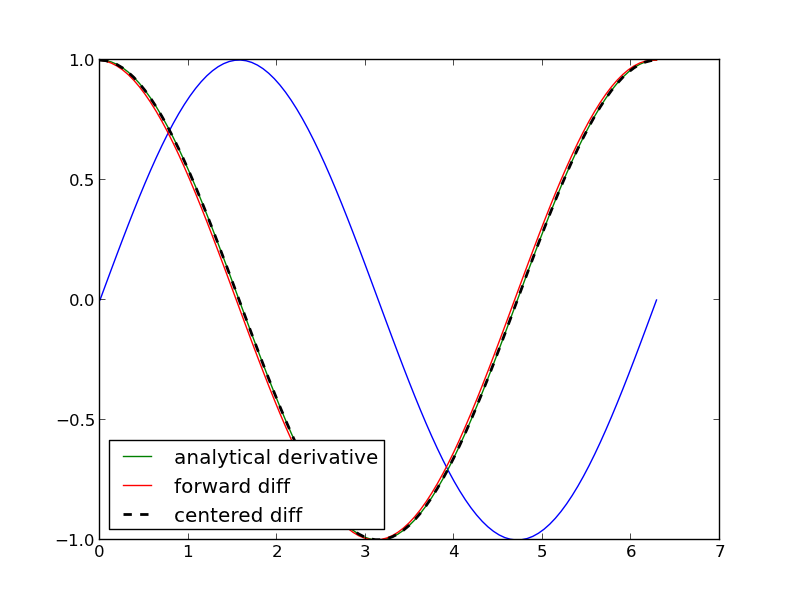## Vectorized numeric derivatives

| categories: math | tags:

Loops are usually not great for performance. Numpy offers some vectorized methods that allow us to compute derivatives without loops, although this comes at the mental cost of harder to understand syntax

import numpy as np
import matplotlib.pyplot as plt

x = np.linspace(0, 2 * np.pi, 100)
y = np.sin(x)
dy_analytical = np.cos(x)

# we need to specify the size of dy ahead because diff returns
#an array of n-1 elements
dy = np.zeros(y.shape, np.float) #we know it will be this size
dy[0:-1] = np.diff(y) / np.diff(x)
dy[-1] = (y[-1] - y[-2]) / (x[-1] - x[-2])

'''
calculate dy by center differencing using array slices
'''

dy2 = np.zeros(y.shape,np.float) #we know it will be this size
dy2[1:-1] = (y[2:] - y[0:-2]) / (x[2:] - x[0:-2])

# now the end points
dy2 = (y - y) / (x - x)
dy2[-1] = (y[-1] - y[-2]) / (x[-1] - x[-2])

plt.plot(x,y)
plt.plot(x,dy_analytical,label='analytical derivative')
plt.plot(x,dy,label='forward diff')
plt.plot(x,dy2,'k--',lw=2,label='centered diff')
plt.legend(loc='lower left')
plt.savefig('images/vectorized-diffs.png')
plt.show()